##### Physics I For Dummies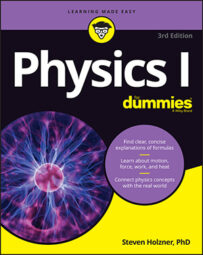In physics terms, acceleration, a, is the amount by which your velocity changes in a given amount of time. Given the initial and final velocities, vi and vf, and the initial and final times over which your speed changes, ti and tf, you can write the equation like this:

In terms of units, the equation looks like this:

Distance per time squared? Don’t let that throw you. You end up with time squared in the denominator because you divide velocity by time. In other words, acceleration is the rate at which your velocity changes, because rates have time in the denominator. For acceleration, you see units of meters per second2, centimeters per second2, miles per second2, feet per second2, or even kilometers per hour2.

It may be easier, for a given problem, to use units such as mph/s (miles per hour per second). This would be useful if the velocity in question had a magnitude of something like several miles per hour that changed typically over a number of seconds.

Say you become a drag racer in order to analyze your acceleration down the dragway. After a test race, you know the distance you went — 402 meters, or about 0.25 miles (the magnitude of your displacement) — and you know the time it took — 5.5 seconds. So what was your acceleration as you blasted down the track?

Well, you can relate displacement, acceleration, and time as follows: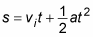and that’s what you want — you always work the algebra so that you end up relating all the quantities you know to the one quantity you don’t know. In this case, you have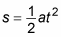(Keep in mind that in this case, your initial velocity is 0 — you’re not allowed to take a running start at the drag race!) You can rearrange this equation with a little algebra to solve for acceleration; just divide both sides by t2 and multiply by 2 to get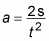Great. Plugging in the numbers, you get the following: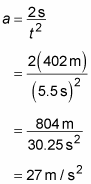Okay, the acceleration is approximately 27 meters per second2. What’s that in more understandable terms? The acceleration due to gravity, g, is 9.8 meters per second2, so this is about 2.7 g’s — you’d feel yourself pushed back into your seat with a force about 2.7 times your own weight.